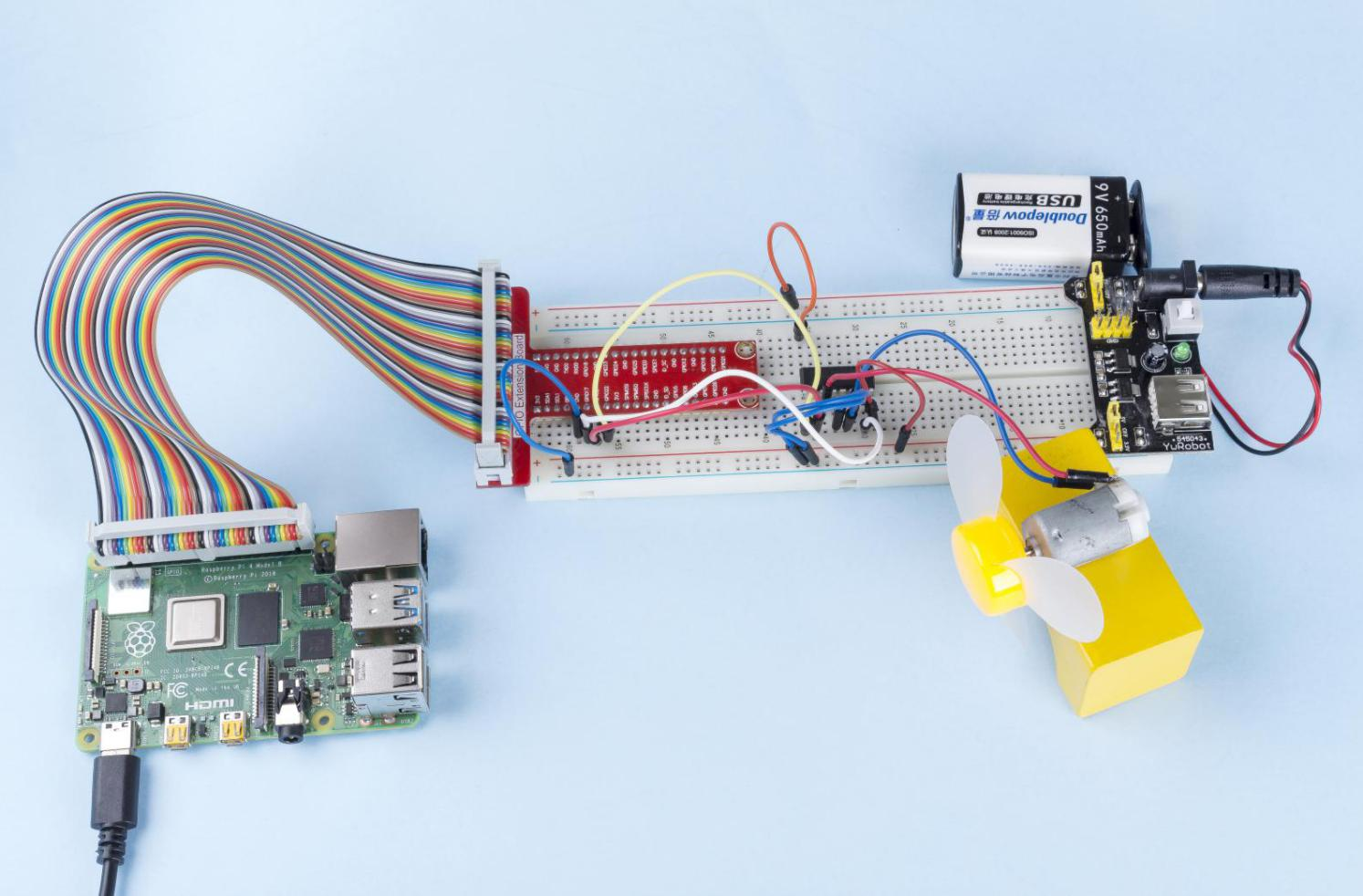# 1.3.1 Motor¶

## Introduction¶

In this lesson, we will learn to how to use L293D to drive a DC motor and make it rotate clockwise and counterclockwise. Since the DC Motor needs a larger current, for safety purpose, here we use the Power Supply Module to supply motors.

## Components¶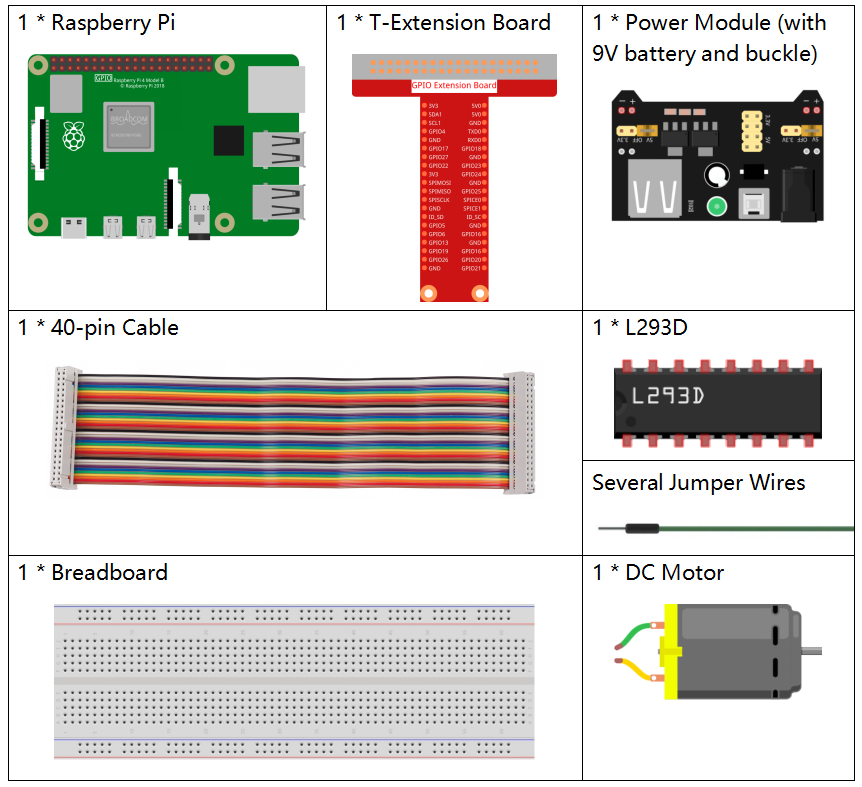## Principle¶

L293D

L293D is a 4-channel motor driver integrated by chip with high voltage and high current. It’s designed to connect to standard DTL, TTL logic level, and drive inductive loads (such as relay coils, DC, Stepper Motors) and power switching transistors etc. DC Motors are devices that turn DC electrical energy into mechanical energy. They are widely used in electrical drive for their superior speed regulation performance.

See the figure of pins below. L293D has two pins (Vcc1 and Vcc2) for power supply. Vcc2 is used to supply power for the motor, while Vcc1 to supply for the chip. Since a small-sized DC motor is used here, connect both pins to +5V.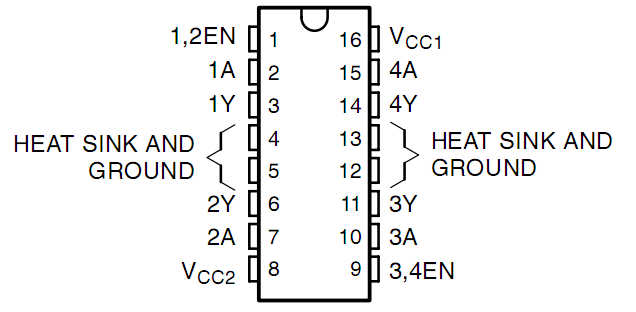The following is the internal structure of L293D. Pin EN is an enable pin and only works with high level; A stands for input and Y for output. You can see the relationship among them at the right bottom. When pin EN is High level, if A is High, Y outputs high level; if A is Low, Y outputs Low level. When pin EN is Low level, the L293D does not work.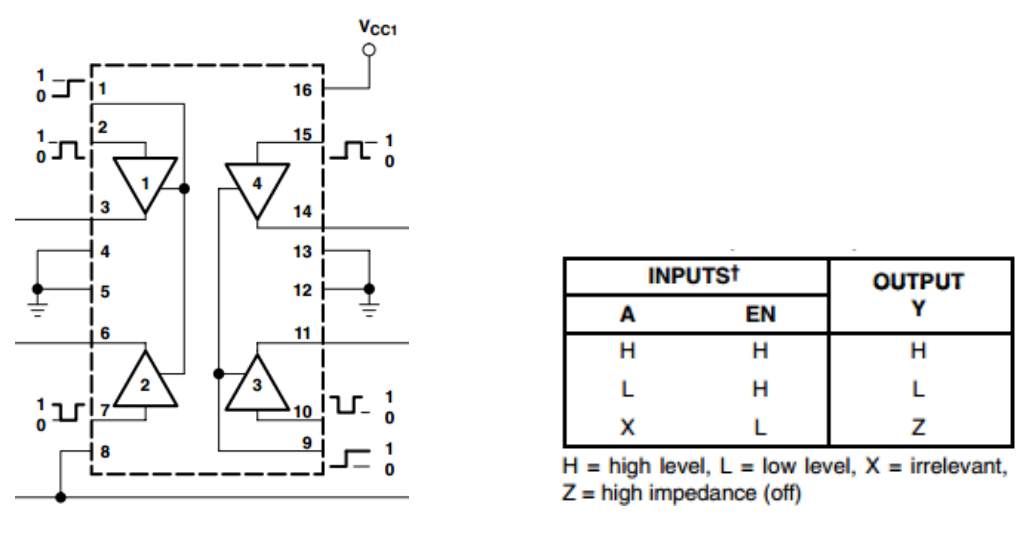DC Motor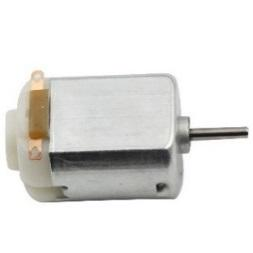This is a 5V DC motor. It will rotate when you give the two terminals of the copper sheet one high and one low level. For convenience, you can weld the pins to it.Power Supply Module

In this experiment, it needs large currents to drive the motor especially when it starts and stops, which will severely interfere with the normal work of Raspberry Pi. Therefore, we separately supply power for the motor by this module to make it run safely and steadily.

You can just plug it in the breadboard to supply power. It provides a voltage of 3.3V and 5V, and you can connect either via a jumper cap included.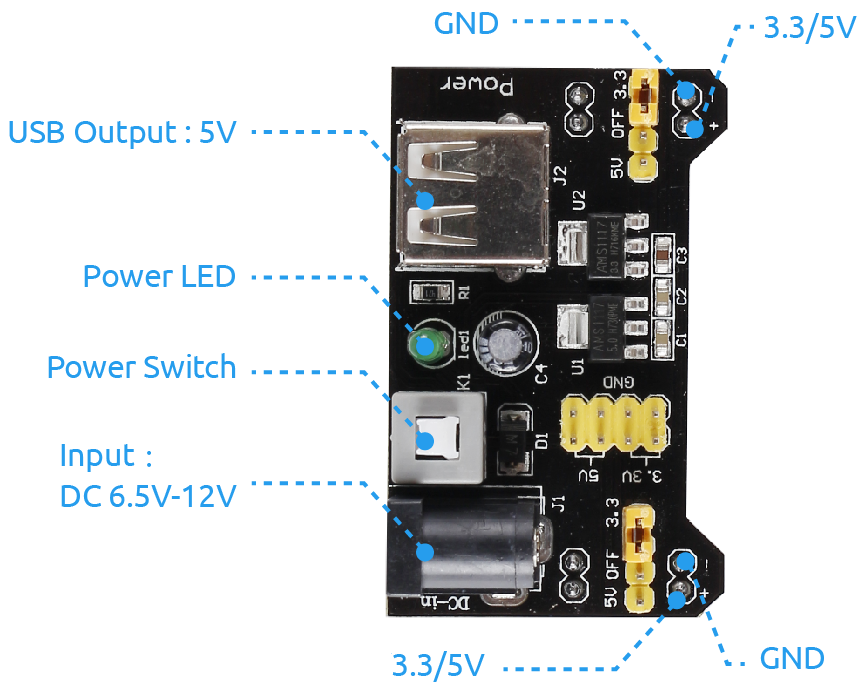## Schematic Diagram¶

Plug the power supply module in breadboard, and insert the jumper cap to pin of 5V, then it will output voltage of 5V. Connect pin 1 of L293D to GPIO22, and set it as high level. Connect pin2 to GPIO27, and pin7 to GPIO17, then set one pin high, while the other low. Thus you can change the motor’s rotation direction.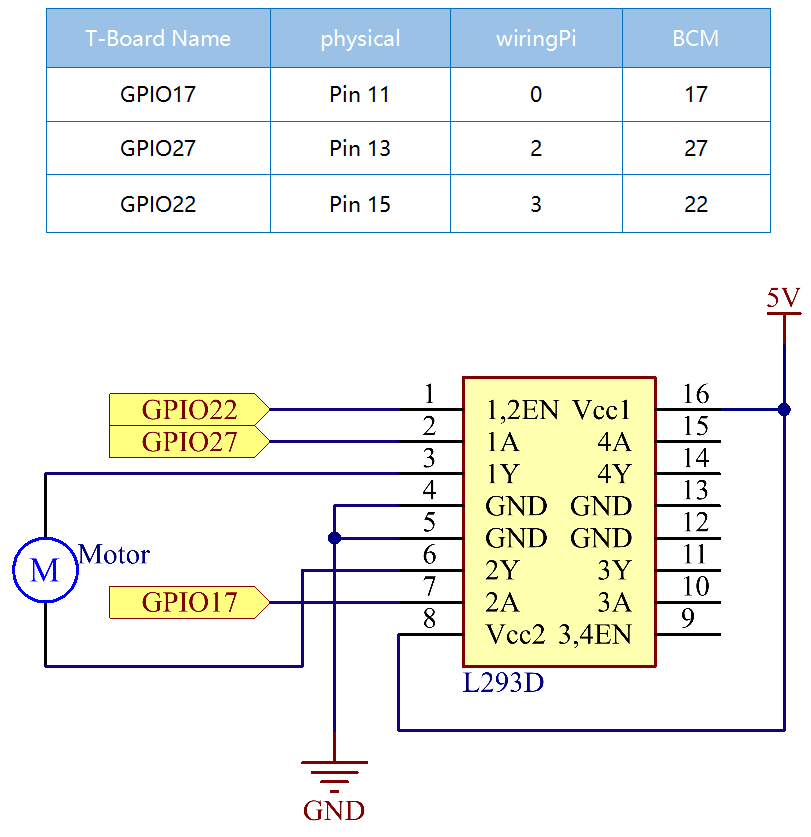## Experimental Procedures¶

Step 1: Build the circuit.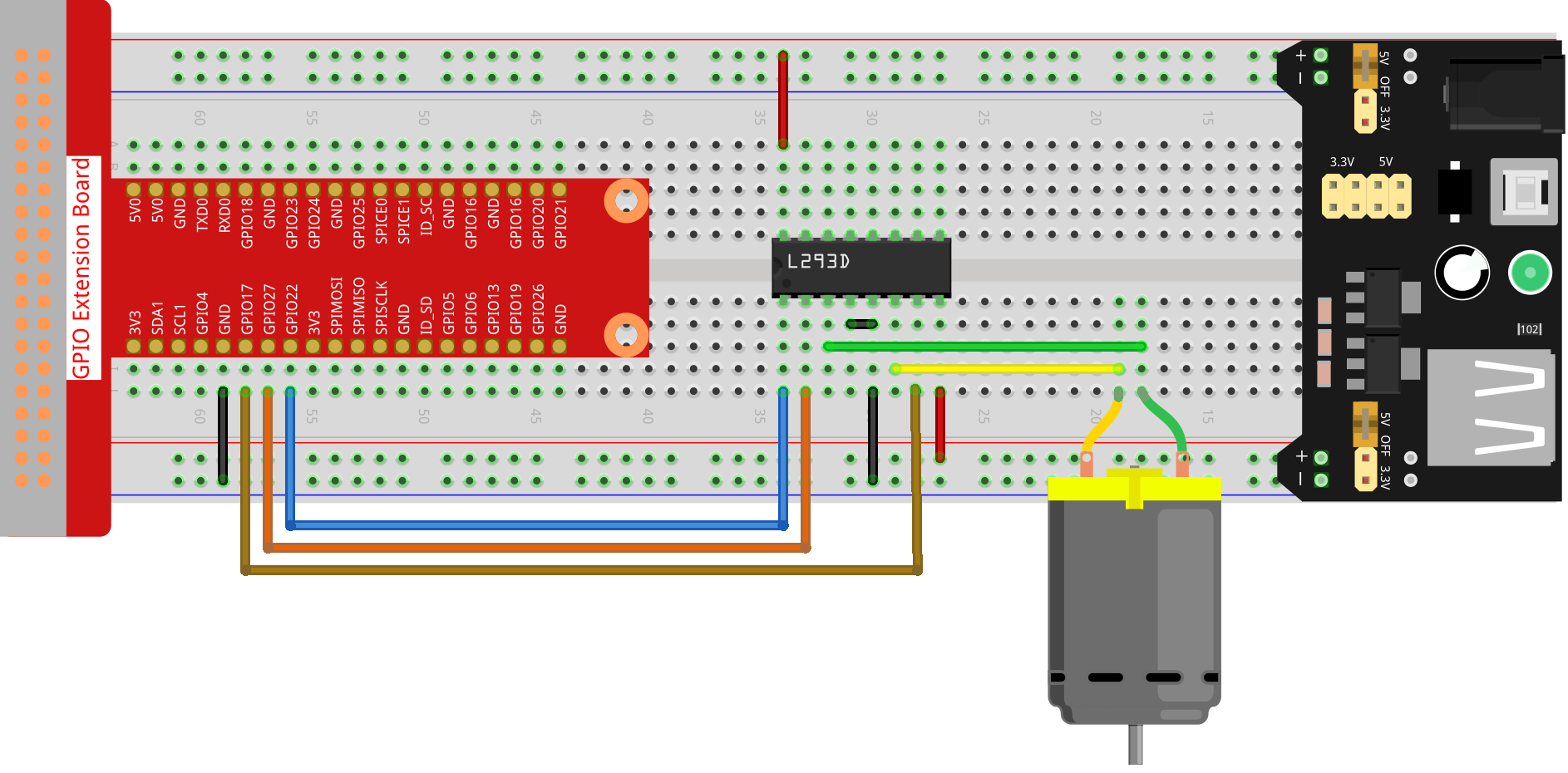Note

The power module can apply a 9V battery with the 9V Battery Buckle in the kit. Insert the jumper cap of the power module into the 5V bus strips of the breadboard.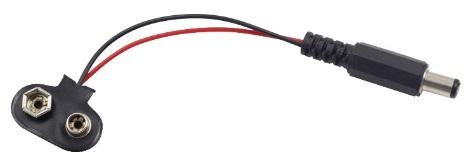### For C Language Users¶

Step 2: Get into the folder of the code.

```cd /home/pi/davinci-kit-for-raspberry-pi/c/1.3.1/
```

Step 3: Compile.

```gcc 1.3.1_Motor.c -lwiringPi
```

Step 4: Run the executable file above.

```sudo ./a.out
```

As the code runs, the motor first rotates clockwise for 5s then stops for 5s, after that, it rotates anticlockwise for 5s; subsequently, the motor stops for 5s. This series of actions will be executed repeatedly.

Note

If it does not work after running, or there is an error prompt: “wiringPi.h: No such file or directory”, please refer to C code is not working?.

Code

```#include <wiringPi.h>
#include <stdio.h>

#define MotorPin1       0
#define MotorPin2       2
#define MotorEnable     3

int main(void){
int i;
if(wiringPiSetup() == -1){ //when initialize wiring failed, print messageto screen
printf("setup wiringPi failed !");
return 1;
}

pinMode(MotorPin1, OUTPUT);
pinMode(MotorPin2, OUTPUT);
pinMode(MotorEnable, OUTPUT);
while(1){
printf("Clockwise\n");
delay(100);
digitalWrite(MotorEnable, HIGH);
digitalWrite(MotorPin1, HIGH);
digitalWrite(MotorPin2, LOW);
for(i=0;i<3;i++){
delay(1000);
}

printf("Stop\n");
delay(100);
digitalWrite(MotorEnable, LOW);
for(i=0;i<3;i++){
delay(1000);
}

printf("Anti-clockwise\n");
delay(100);
digitalWrite(MotorEnable, HIGH);
digitalWrite(MotorPin1, LOW);
digitalWrite(MotorPin2, HIGH);
for(i=0;i<3;i++){
delay(1000);
}

printf("Stop\n");
delay(100);
digitalWrite(MotorEnable, LOW);
for(i=0;i<3;i++){
delay(1000);
}
}
return 0;
}
```

Code Explanation

```digitalWrite(MotorEnable, HIGH);
```

Enable the L239D.

```digitalWrite(MotorPin1, HIGH);
digitalWrite(MotorPin2, LOW);
```

Set a high level for 2A(pin 7); since 1,2EN(pin 1) is in high level, 2Y will output high level.

Set a low level for 1A, then 1Y will output low level, and the motor will rotate.

```for(i=0;i<3;i++){
delay(1000);
}
```

this loop is to delay for 3*1000ms.

```digitalWrite(MotorEnable, LOW)
```

If 1,2EN (pin1) is in low level, L293D does not work. Motor stops rotating.

```digitalWrite(MotorPin1, LOW)
digitalWrite(MotorPin2, HIGH)
```

Reverse the current flow of the motor, then the motor will rotate reversely.

### For Python Language Users¶

Step 2: Get into the folder of the code.

```cd /home/pi/davinci-kit-for-raspberry-pi/python
```

Step 3: Run.

```sudo python3 1.3.1_Motor.py
```

As the code runs, the motor first rotates clockwise for 5s then stops for 5s, after that, it rotates anticlockwise for 5s; subsequently, the motor stops for 5s. This series of actions will be executed repeatedly.

Code

Note

You can Modify/Reset/Copy/Run/Stop the code below. But before that, you need to go to source code path like `davinci-kit-for-raspberry-pi/python`.

```import RPi.GPIO as GPIO
import time

dhtPin = 17

GPIO.setmode(GPIO.BCM)

MAX_UNCHANGE_COUNT = 100

STATE_INIT_PULL_DOWN = 1
STATE_INIT_PULL_UP = 2
STATE_DATA_FIRST_PULL_DOWN = 3
STATE_DATA_PULL_UP = 4
STATE_DATA_PULL_DOWN = 5

GPIO.setup(dhtPin, GPIO.OUT)
GPIO.output(dhtPin, GPIO.HIGH)
time.sleep(0.05)
GPIO.output(dhtPin, GPIO.LOW)
time.sleep(0.02)
GPIO.setup(dhtPin, GPIO.IN, GPIO.PUD_UP)

unchanged_count = 0
last = -1
data = []
while True:
current = GPIO.input(dhtPin)
data.append(current)
if last != current:
unchanged_count = 0
last = current
else:
unchanged_count += 1
if unchanged_count > MAX_UNCHANGE_COUNT:
break

state = STATE_INIT_PULL_DOWN

lengths = []
current_length = 0

for current in data:
current_length += 1

if state == STATE_INIT_PULL_DOWN:
if current == GPIO.LOW:
state = STATE_INIT_PULL_UP
else:
continue
if state == STATE_INIT_PULL_UP:
if current == GPIO.HIGH:
state = STATE_DATA_FIRST_PULL_DOWN
else:
continue
if state == STATE_DATA_FIRST_PULL_DOWN:
if current == GPIO.LOW:
state = STATE_DATA_PULL_UP
else:
continue
if state == STATE_DATA_PULL_UP:
if current == GPIO.HIGH:
current_length = 0
state = STATE_DATA_PULL_DOWN
else:
continue
if state == STATE_DATA_PULL_DOWN:
if current == GPIO.LOW:
lengths.append(current_length)
state = STATE_DATA_PULL_UP
else:
continue
if len(lengths) != 40:
#print ("Data not good, skip")
return False

shortest_pull_up = min(lengths)
longest_pull_up = max(lengths)
halfway = (longest_pull_up + shortest_pull_up) / 2
bits = []
the_bytes = []
byte = 0

for length in lengths:
bit = 0
if length > halfway:
bit = 1
bits.append(bit)
#print ("bits: %s, length: %d" % (bits, len(bits)))
for i in range(0, len(bits)):
byte = byte << 1
if (bits[i]):
byte = byte | 1
else:
byte = byte | 0
if ((i + 1) % 8 == 0):
the_bytes.append(byte)
byte = 0
#print (the_bytes)
checksum = (the_bytes + the_bytes + the_bytes + the_bytes) & 0xFF
if the_bytes != checksum:
#print ("Data not good, skip")
return False

return the_bytes, the_bytes

def main():

while True:
if result:
humidity, temperature = result
print ("humidity: %s %%,  Temperature: %s C`" % (humidity, temperature))
time.sleep(1)

def destroy():
GPIO.cleanup()

if __name__ == '__main__':
try:
main()
except KeyboardInterrupt:
destroy()
```

Code Explanation

```def motor(direction):
# Clockwise
if direction == 1:
# Set direction
GPIO.output(MotorPin1, GPIO.HIGH)
GPIO.output(MotorPin2, GPIO.LOW)
# Enable the motor
GPIO.output(MotorEnable, GPIO.HIGH)
print ("Clockwise")
...
```

Create a function, motor() whose variable is direction. As the condition that direction=1 is met, the motor rotates clockwise; when direction=-1, the motor rotates anticlockwise; and under the condition that direction=0, it stops rotating.

```def main():
# Define a dictionary to make the script more readable
# CW as clockwise, CCW as counterclockwise, STOP as stop
directions = {'CW': 1, 'CCW': -1, 'STOP': 0}
while True:
# Clockwise
motor(directions['CW'])
time.sleep(5)
# Stop
motor(directions['STOP'])
time.sleep(5)
# Anticlockwise
motor(directions['CCW'])
time.sleep(5)
# Stop
motor(directions['STOP'])
time.sleep(5)
```

In the main（）function, create an array, directions[], in which CW is equal to 1, the value of CCW is -1, and the number 0 refers to Stop.

As the code runs, the motor first rotates clockwise for 5s then stop for 5s, after that, it rotates anticlockwise for 5s; subsequently, the motor stops for 5s. This series of actions will be executed repeatedly.

Now, you should see the motor blade rotating.

## Phenomenon Picture¶